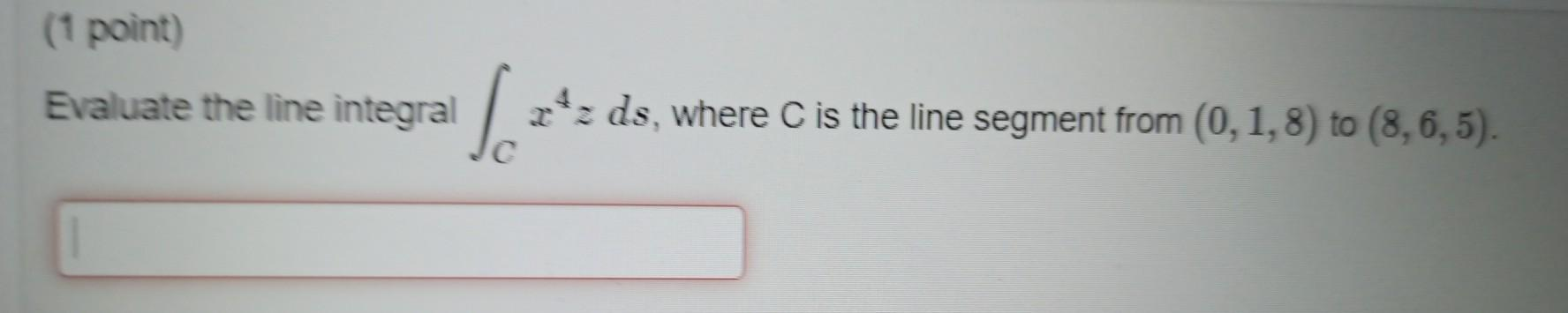### Create an Account

Already have account?

### Forgot Your Password ?

Home / Questions / (1 point) Evaluate the line integral x+ds, where C is the line segment from (0,1, 8) to (8,6,5).

# (1 point) Evaluate the line integral x+ds, where C is the line segment from (0,1, 8) to (8,6,5).

(1 point) Evaluate the line integral x+ds, where C is the line segment from (0,1, 8) to (8,6,5).Apr 15 2021 View more View Less

#### Answer (Solved)Subscribe To Get Solution